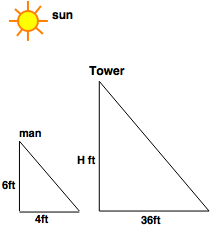# Height and Distance - Aptitude Questions and Answers

## Height and Distance Questions and Answers

Learn and practice the chapter "Height and Distance" with these solved Aptitude Questions and Answers. Each question in the topic is accompanied by a clear and easy explanation, diagrams, formulae, shortcuts and tricks that help in understanding the concept.

## Use of Height and Distance Questions

The questions and examples given in this section will be useful to all the freshers, college students and engineering students preparing for placement tests or any competitive exam like MBA, CAT, MAT, SNAP, MHCET, XAT, NMAT, GATE, Bank exams - IBPS, SBI, RBI, RRB, SSB, SSC, UPSC etc.

Practice with this online test to crack your placements and entrance tests!
1. Mohit looks at the top of a tower. The angle made is 45°. The angle made reduces to 30°, if he moves back by 10 m. How high is the tower?

a. 10/(3 + 1) m
b. 10/(3 - 1) m
c. 20 m
d. 203 m

Answer: b. 10/(3 - 1) m

Explanation:

Let PQ be the tree and M and N be positions where Mohan stands.

Tip:
 Tan θ = P B
Where, P = Perpendicular ; B = Base
 Tan 30° = 1 ; Tan 45° = 1; 3
Tan 60° = 3 ; Tan 90° = Not defined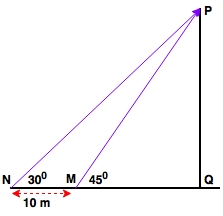Now, Tan 45° = 1 = PQ MQ
∴ PQ = MQ
 Tan 30° = 1 = PQ = PQ 3 NQ 10+MQ
∴ 10 + MQ = 3 PQ
∴ 10 + PQ = 3 PQ --------> As, PQ = MQ
 ∴ PQ = 10 m 3 - 1

2. A street light pole stands between two parallel roads. The height of the pole is 10m. From the top of the tower, the angles of depression of the roads are 45° and 30°. Find the distance between the roads.

a. 10/3m
b. 203m
c. 27.32 m
d. 29.56 m

Explanation:

Tip:
 Tan θ = P B
Where, P = Perpendicular ; B = Base
 Tan 30° = 1 ; Tan 45° = 1; 3
Tan 60° = 3 ; Tan 90° = Not defined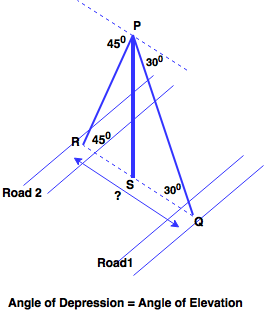Tower PS = 10m in height
 tan 45° = 1 = PS RS
∴ PS = RS = 10
 Tan 30° = 1 = PS = 10 3 SQ SQ
∴ SQ = 103
RQ = RS + SQ = 10 + 103 = 27.32m = distance between 2 roads

3. A tree of height 24 cm stands in my vicinity. On a stormy night, the tree broke and fell in such a way that the upper part remained attached to the stem, forming an angle of 30° with the ground. Find the height from where it broke.

a. 7.5 cm
b. 8 cm
c. 9.5 cm
d. 12 cm

Explanation:

Let the tree break at height h cm from ground at point M.
The broken part makes angle of 30°
∴ Broken Part MP = MN = 24 - h (Orange line in diagram)

Tip:
 Sin θ = P H
Where, P = Perpendicular ;
H = Hypotenuse
 Sin 30° = 1 ; Sin 45° = 1 ; 2 2
 Sin 60° = 3 ; Sin 90° = 1 2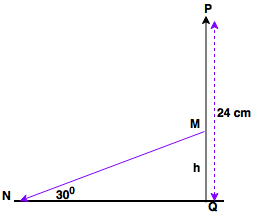In △MNQ,
 Sin 30° = 1 = MQ = h 2 MN 24-h
∴ 24 - h = 2h
∴ h = 8cm = Tree breaks at this height

4. The shadow formed by a man on a sunny day is 1/3 times the actual height. Find the sun's angle of elevation?

a. 30°
b. 45°
c. 60°
d. 135°

Explanation:

Tip:
 Tan θ = P B
Where, P = Perpendicular ; B = Base
 Tan 30° = 1 ; Tan 45° = 1; 3
Tan 60° = 3 ; Tan 90° = Not defined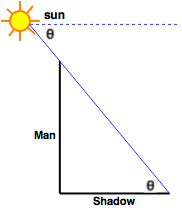Shadow Length = 1 Height of man 3
 Height of man = 3 Shadow length
 Tan θ = Height of man = 3 Shadow length
But, Tan 60° = 3
∴ θ = 60° = Angle of elevation of sun

5. The actual height of a man is 6 feet but due to the position of Sun he casts a shadow of 4 feet. He is standing next to an electricity tower and notices that it casts a shadow of 36 feet. Find the height of the electricity tower.

a. 48 ft
b. 54 ft
c. 63 ft
d. 72 ft